Simulations of Some Random Fields

 α=0.3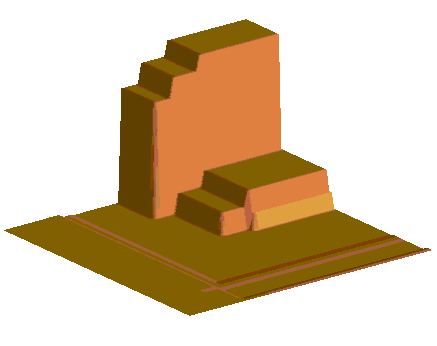α=0.5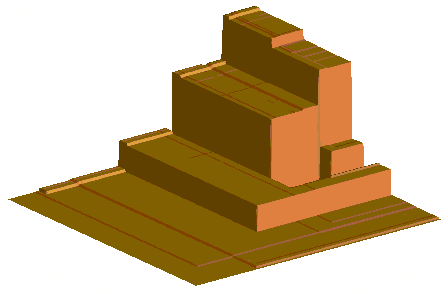α=0.7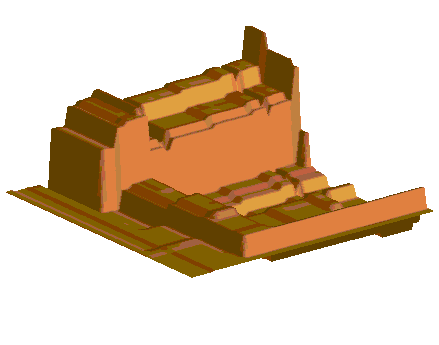α=1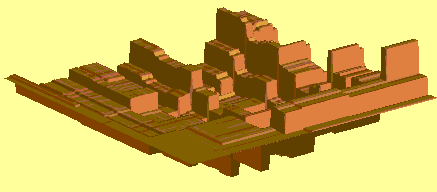α=1.1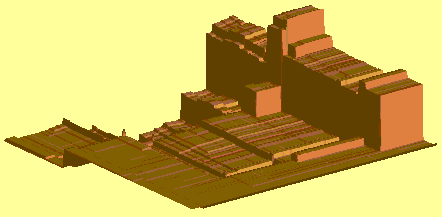α=1.5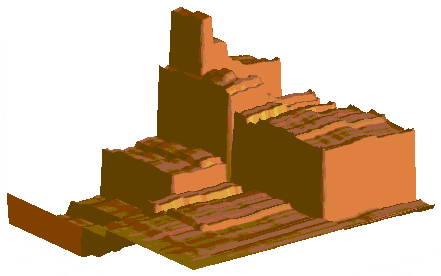α=1.7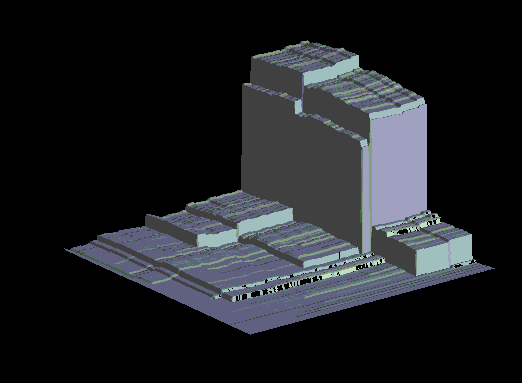α=1.8α=1.9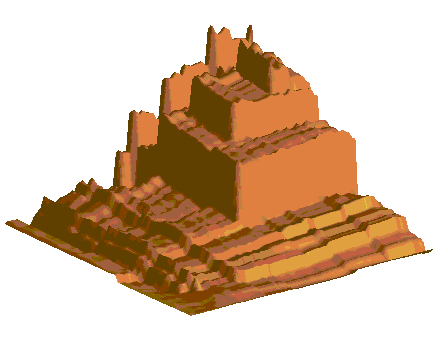α=2.0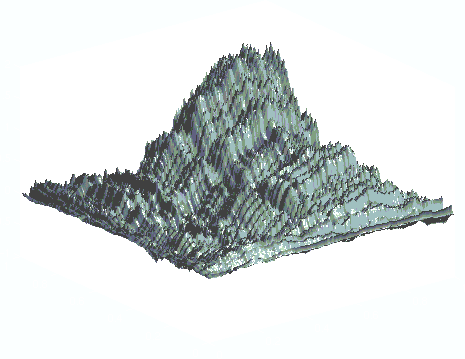α=1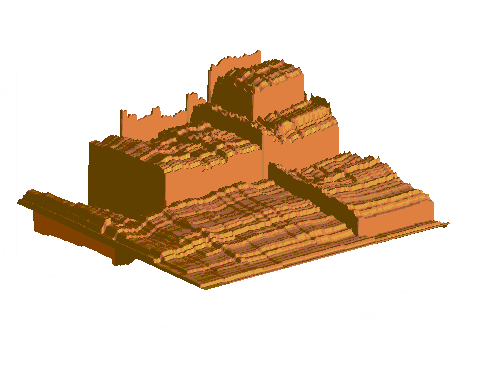α=1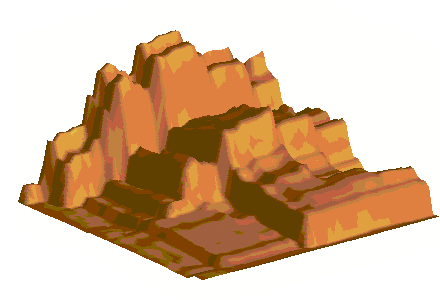α=1.9α=2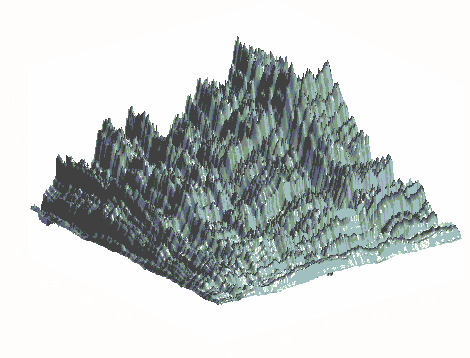α=2α=2An avi movie of dynamical random walk, scaled to simulate the Ornstein-Uhlenbeck process on Wiener space. (1.35 GB!) This movie uses MatLab software written in 2004 by David A. Levin. Its little cousin is smaller (67.3 MB).

155 South 1400 East, Room 102, Salt Lake City, UT 84112-0090, Tel:+1 801 581 3896, Fax:+1 801 581 4148